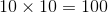# GRE Subject Test: Biology : Understanding Light Microscopy

## Example Questions

### Example Question #1 : Microscopy

To what power would a microscope magnify an object if the 10x low-power objective were in place and the microscope has a standard eyepiece?

10x

1x

100x

1000x

10000x

100x

Explanation:

The occular, or eye piece, has a magnification power of 10x. If the 10x low-power objective were in place, the magnification would be multiplicative.### Example Question #2 : Understanding Light Microscopy

Which term is used to describe the smallest structure that can be seen by a light microscope?

Resolving power

Visual acuity

Index of refraction

Optical transmission

Optotype

Resolving power

Explanation:

Optical transmission is the amount of light that passes through a laboratory specimen. It can be used to measure the concentration of components in the specimen. Optotype is the variable-sized type used in testing visual acuity. Visual acuity is a measure of the resolving power of the eye; the smallest type that can be seen at a distance. The index of refraction is defined as the speed of light in a vacuum divided by the speed of light in a medium.

The resolving power of a light microscope is the smallest structure that can be seen, approximately 1 micrometer (a millionth of a meter) by the microscope.

### Example Question #1 : Lab Techniques

When using a light microscope, the light waves that pass through or bounce off of a specimen are focused and magnified by which of the following?

Occulars

Diaphgram

Iris

Fine focus

Lens